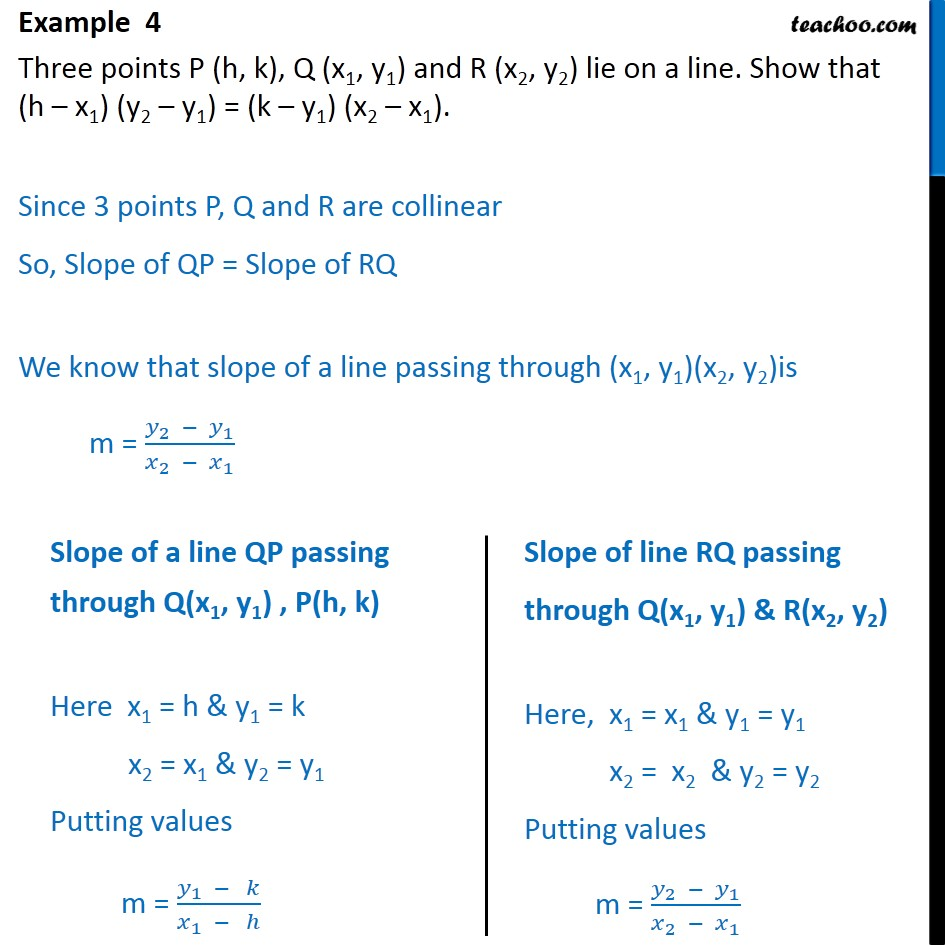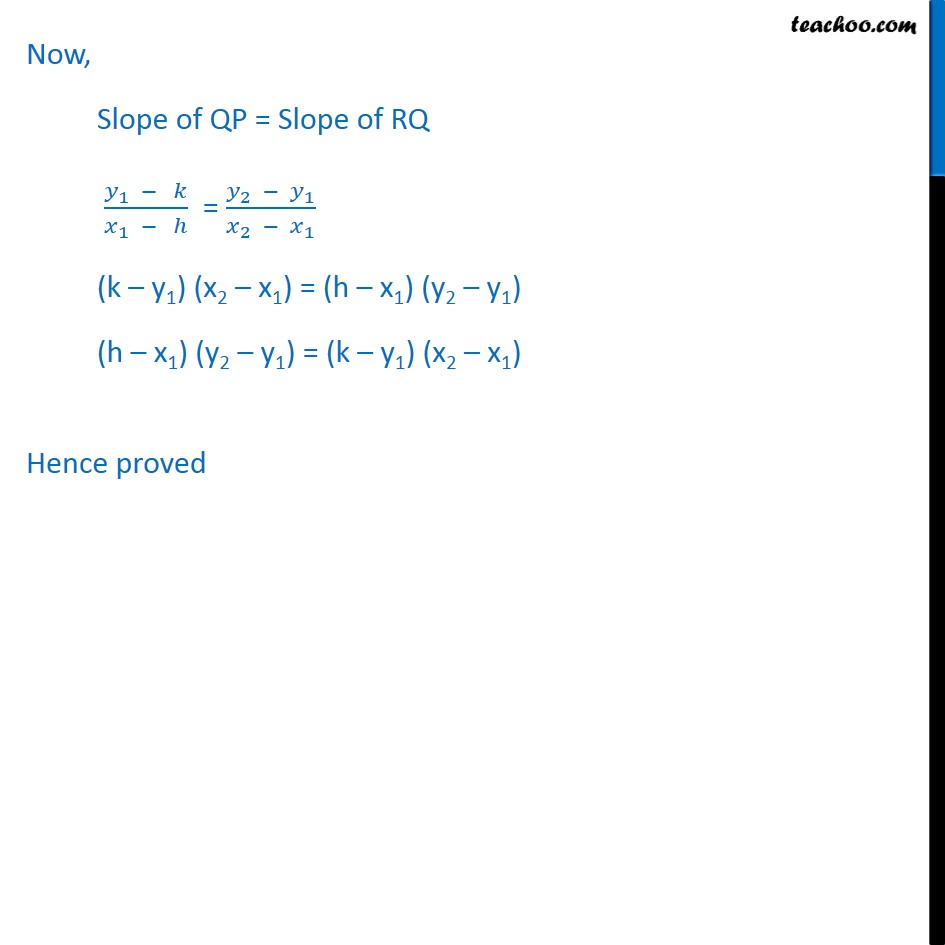Examples

Chapter 9 Class 11 Straight Lines
Serial order wiseLearn in your speed, with individual attention - Teachoo Maths 1-on-1 Class

### Transcript

Example 4 Three points P (h, k), Q (x1, y1) and R (x2, y2) lie on a line. Show that (h x1) (y2 y1) = (k y1) (x2 x1). Since 3 points P, Q and R are collinear So, Slope of QP = Slope of RQ We know that slope of a line passing through (x1, y1)(x2, y2)is m = ( _2 _1)/( _2 _1 ) Now, Slope of QP = Slope of RQ ( _1 )/( _1 ) = ( _2 _1)/( _2 _1 ) (k y1) (x2 x1) = (h x1) (y2 y1) (h x1) (y2 y1) = (k y1) (x2 x1) Hence proved